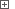# Sorting Data in Syncfusion GridDataBoundGridThe data displayed by the GridDataBoundGrid control can be sorted by a particular column. Typically, users can sort the grid data and change the sort direction (ascending or descending) by clicking or double-clicking the column header. However, implementing this functionality in scripts is a difficult task and we will not consider it here. Below, we will explain how to perform sorting using internal methods of the GridDataBoundGrid control.

To sort data in the GridDataBoundGrid control, you can use the `SortColumn` method:

GridObj.SortColumn( ColIndex )

This method takes the index of the desired column as a parameter. The subsequent call of this method reverses the sort direction. Sorting the grid data by a particular column automatically cancels sorting by other columns.

To determine whether the grid is sorted by a particular column, you can use the `HasTag` and `Tag` properties of the column header cell (the cell in the row with the index of 0 and the given column). If the grid is sorted by the given column, the `HasTag` property returns True and the `Tag` property holds the value indicating the sort direction (ascending or descending). If the column does not participate in sorting, the `HasTag` property returns False and the `Tag` property returns the empty value (`null` in JavaScript, JScript, C#Script and C++Script, `None` in Python, `Nothing` in VBScript, `nil` in DelphiScript)

 Note: In order for TestComplete to access the mentioned properties and methods of the GridDataBoundGrid control and its helper objects, the .NET Application Support plugin must be installed and enabled.

Below is an example that illustrates how you can use this method to sort data in the GridDataBoundGrid control.

###### ExampleView description

JavaScript, JScript

function Main ()
{
var p, Grid;

// Obtain the application process and the grid object
p = Sys.Process("DataBoundSortByDisplayMember");
Grid = p.WinFormsObject("Form1").WinFormsObject("gridDataBoundGrid1");

// Sort data by the "ProductName" column
Sort (Grid, "ProductName", "Ascending");
aqUtils.Delay (1000);

// Sort data by the "Supplier" column
Sort (Grid, "SupplierID", "Descending");
}

function Sort (Grid, ColumnId, SortOrder)
{
// Get the column's absolute index
var ColIndex = GetColIndexById (Grid, ColumnId);

// Check if the column is already sorted
if (Grid.Item(0, ColIndex).HasTag)
{
// If the sort direction differs from the specified one...
if (Grid.Item(0, ColIndex).Tag.OleValue != SortOrder)
// ... then toggle the sort direction
Grid.SortColumn (ColIndex);
}
else
{
// Sort in the specified direction
Grid.SortColumn (ColIndex);   // Ascending
if (SortOrder == "Descending")
Grid.SortColumn (ColIndex); // Descending
}
}

function GetColIndexById (Grid, ColumnId)
{
if (aqObject.GetVarType(ColumnId) == varOleStr)
return Grid.NameToColIndex(ColumnId)
else
return ColumnId;
}

Python

``````def Main ():

# Obtain the application process and the grid object
p = Sys.Process("DataBoundSortByDisplayMember");
Grid = p.WinFormsObject("Form1").WinFormsObject("gridDataBoundGrid1");

# Sort data by the "ProductName" column
Sort (Grid, "ProductName", "Ascending");
aqUtils.Delay (1000);

# Sort data by the "Supplier" column
Sort (Grid, "SupplierID", "Descending");

def Sort (Grid, ColumnId, SortOrder):
# Get the column's absolute index
ColIndex = GetColIndexById (Grid, ColumnId);

# Check if the column is already sorted
if Grid.Item[0, ColIndex].HasTag:
# If the sort direction differs from the specified one...
if (Grid.Item[0, ColIndex].Tag.OleValue != SortOrder):
# ... then toggle the sort direction
Grid.SortColumn (ColIndex);
else:
# Sort in the specified direction
Grid.SortColumn (ColIndex);   # Ascending
if (SortOrder == "Descending"):
Grid.SortColumn (ColIndex); # Descending

def GetColIndexById (Grid, ColumnId):
if (aqObject.GetVarType(ColumnId) == varOleStr):
return Grid.NameToColIndex(ColumnId)
else:
return ColumnId;``````

VBScript

Sub Main
Dim p, Grid

' Obtain the application process and the grid object
Set p = Sys.Process("DataBoundSortByDisplayMember")
Set Grid = p.WinFormsObject("Form1").WinFormsObject("gridDataBoundGrid1")

' Sort data by the "ProductName" column
Call Sort (Grid, "ProductName", "Ascending")
Call aqUtils.Delay (1000)

' Sort data by the "Supplier" column
Call Sort (Grid, "SupplierID", "Descending")
End Sub

Sub Sort (Grid, ColumnId, SortOrder)
Dim ColIndex

' Get the column's absolute index
ColIndex = GetColIndexById (Grid, ColumnId)

' Check if the column is already sorted
If Grid.Item(0, ColIndex).HasTag Then
' If the sort direction differs from the specified one...
If Grid.Item(0, ColIndex).Tag.OleValue <> SortOrder Then
' ... then toggle the sort direction
Call Grid.SortColumn (ColIndex)
End If
Else
' Sort in the specified direction
Call Grid.SortColumn (ColIndex)   ' Ascending
If SortOrder = "Descending" Then
Call Grid.SortColumn (ColIndex) ' Descending
End If
End If
End Sub

Function GetColIndexById (Grid, ColumnId)
If aqObject.GetVarType(ColumnId) = varOleStr Then
GetColIndexById = Grid.NameToColIndex(ColumnId)
Else
GetColIndexById = ColumnId
End If
End Function

DelphiScript

procedure Sort (Grid, ColumnId, SortOrder); forward;
function GetColIndexById (Grid, ColumnId); forward;

procedure Main;
var p, Grid : OleVariant;
begin
// Obtain the application process and the grid object
p := Sys.Process('DataBoundSortByDisplayMember');
Grid := p.WinFormsObject('Form1').WinFormsObject('gridDataBoundGrid1');

// Sort data by the 'ProductName' column
Sort (Grid, 'ProductName', 'Ascending');
aqUtils.Delay (1000);

// Sort data by the 'Supplier' column
Sort (Grid, 'SupplierID', 'Descending');
end;

procedure Sort (Grid, ColumnId, SortOrder);
var ColIndex : OleVariant;
begin
// Get the column's absolute index
ColIndex := GetColIndexById (Grid, ColumnId);

// Check if the column is already sorted
if Grid.Item[0, ColIndex].HasTag then
begin
// If the sort direction differs from the specified one...
if Grid.Item[0, ColIndex].Tag.OleValue <> SortOrder then
// ... then toggle the sort direction
Grid.SortColumn (ColIndex);
end
else begin
// Sort in the specified direction
Grid.SortColumn (ColIndex);   // Ascending
if SortOrder = 'Descending' then
Grid.SortColumn (ColIndex); // Descending
end
end;

function GetColIndexById (Grid, ColumnId);
begin
if aqObject.GetVarType(ColumnId) = varOleStr then
Result := Grid.NameToColIndex(ColumnId)
else
Result := ColumnId;
end;

C++Script, C#Script

function Main ()
{
var p, Grid;

// Obtain the application process and the grid object
p = Sys["Process"]("DataBoundSortByDisplayMember");
Grid = p["WinFormsObject"]("Form1")["WinFormsObject"]("gridDataBoundGrid1");

// Sort data by the "ProductName" column
Sort (Grid, "ProductName", "Ascending");
aqUtils["Delay"] (1000);

// Sort data by the "Supplier" column
Sort (Grid, "SupplierID", "Descending");
}

function Sort (Grid, ColumnId, SortOrder)
{
// Get the column's absolute index
var ColIndex = GetColIndexById (Grid, ColumnId);

// Check if the column is already sorted
if (Grid["Item"](0, ColIndex)["HasTag"])
{
// If the sort direction differs from the specified one...
if (Grid["Item"](0, ColIndex)["Tag"]["OleValue"] != SortOrder)
// ... then toggle the sort direction
Grid["SortColumn"](ColIndex);
}
else
{
// Sort in the specified direction
Grid["SortColumn"](ColIndex);   // Ascending
if (SortOrder == "Descending")
Grid["SortColumn"](ColIndex); // Descending
}
}

function GetColIndexById (Grid, ColumnId)
{
if (aqObject["GetVarType"](ColumnId) == varOleStr)
return Grid["NameToColIndex"](ColumnId)
else
return ColumnId;
}